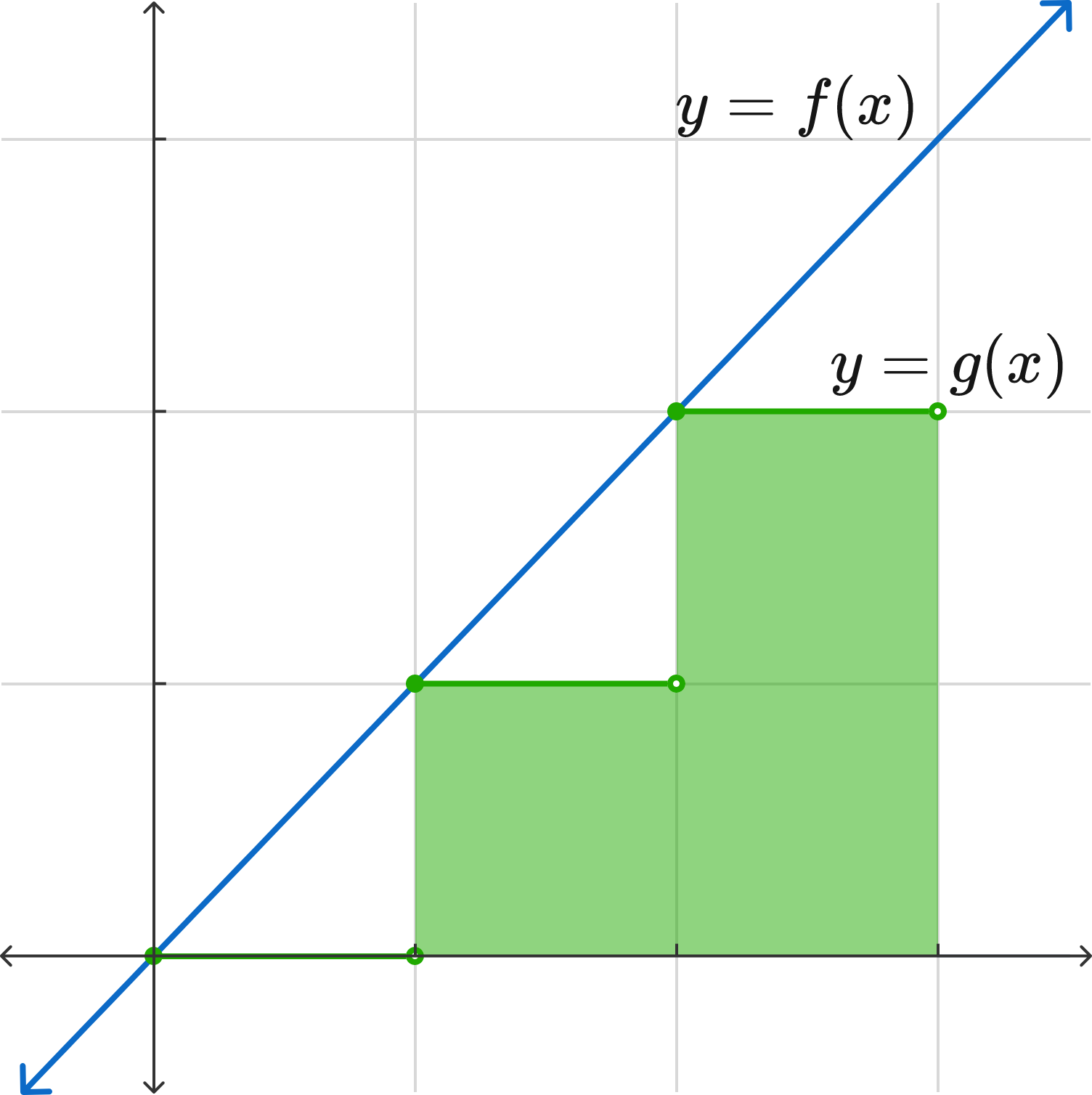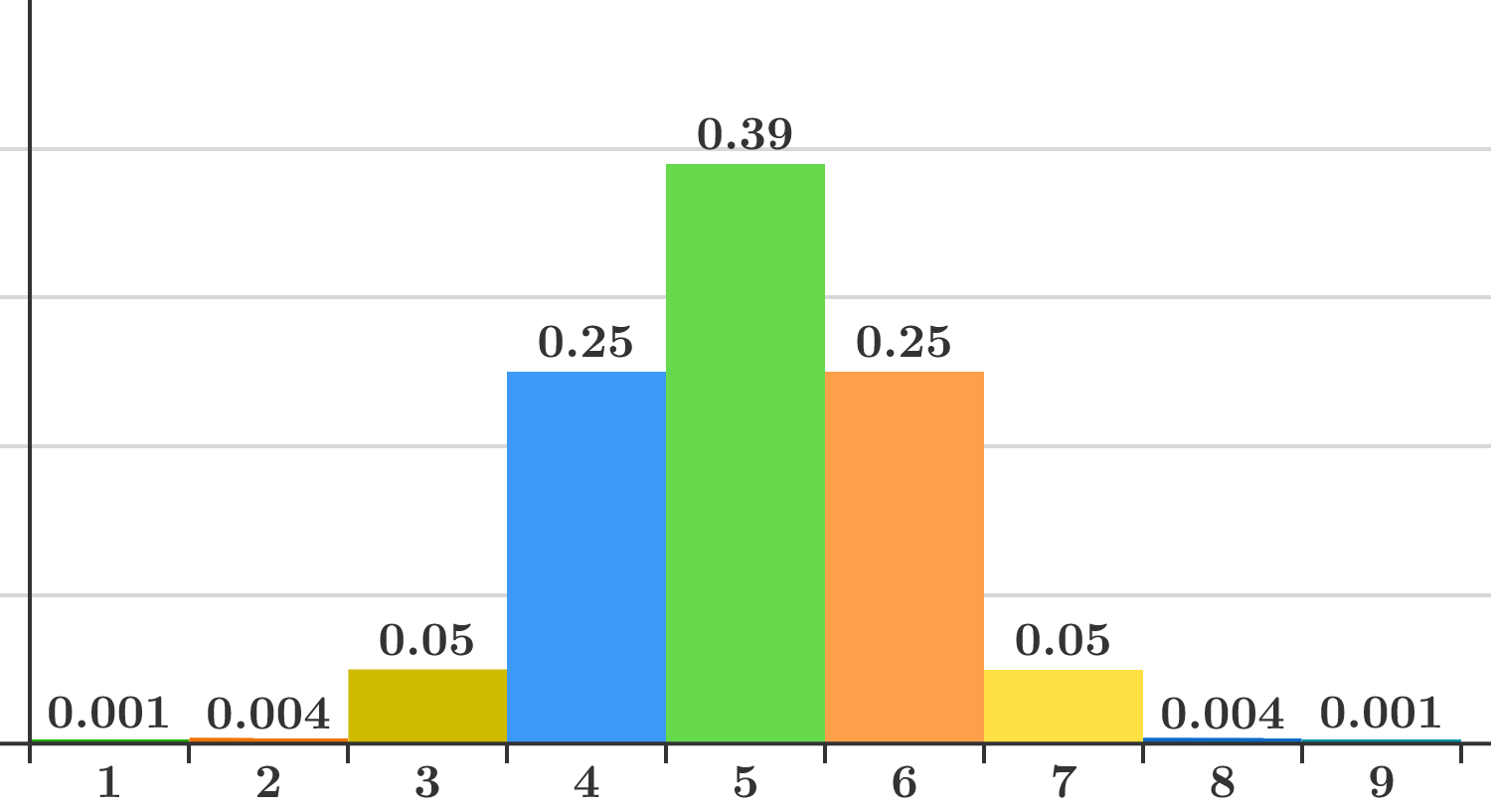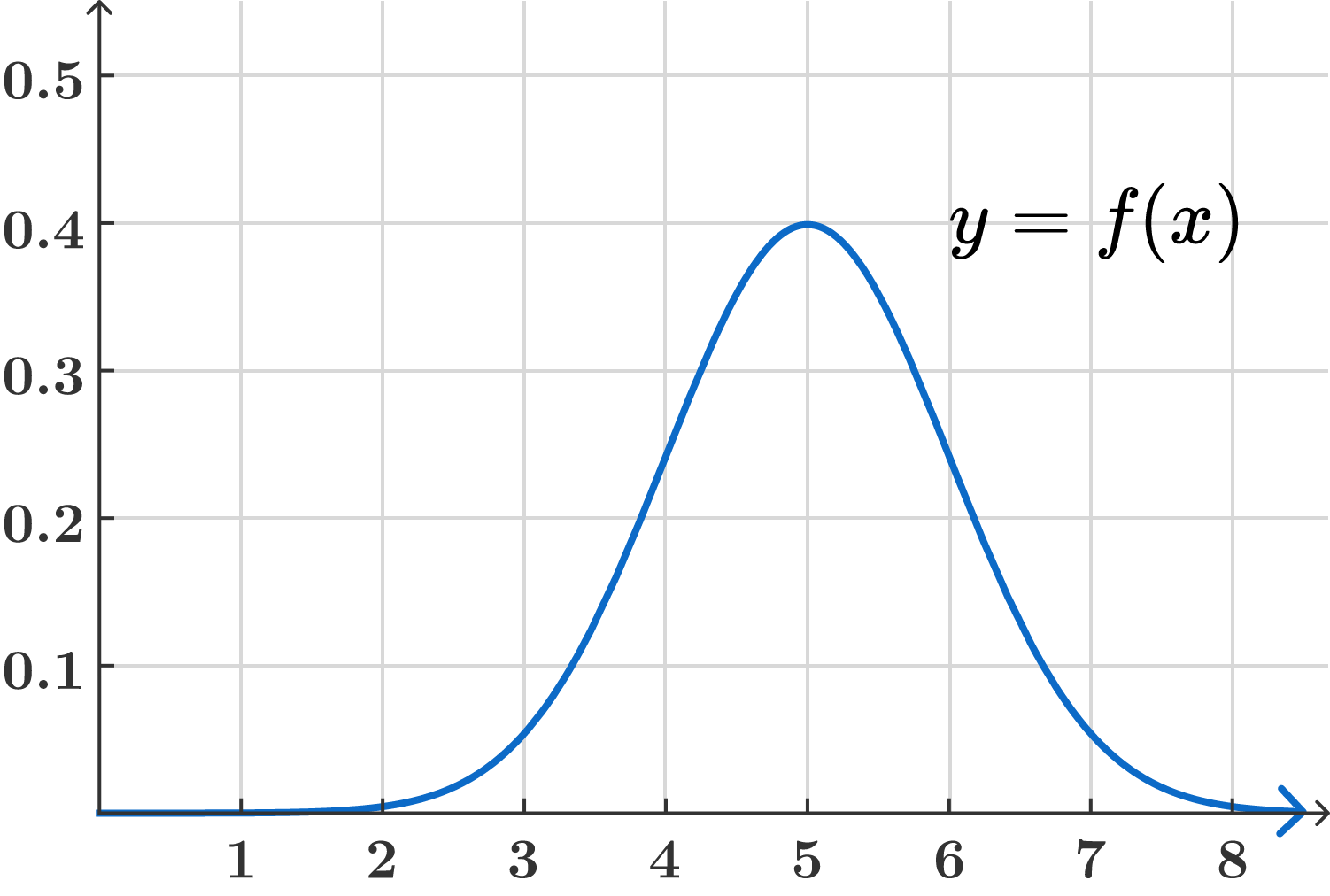### Integral Calculus

In this quiz, we’ll take a look at how Riemann sums naturally appear in a variety of problems. Let’s start by working with the average value of a function.

Let $f(x)$ be the following piecewise function:

$f(x) = \begin{cases} 1 & 0 \leq x \leq \frac{1}{3} \\ 10 & \frac{1}{3} < x \leq 1.\end{cases}$

What is the average value of $f(x)$ over the interval $[0,1]?$

# Different Problem, Same Idea

Let $f(x) = \lfloor x \rfloor$, meaning the largest integer not exceeding $x.$ For example, $f(\pi) = 3, f(5.99) = 5$, and $f(6) = 6.$ What is the average value of $f(x)$ on the interval $[0,10)?$

# Different Problem, Same Idea

We’ve now seen that calculating the average value of step functions (piecewise functions where each segment maps to a constant) is relatively straightforward. We multiply the value of $f$ on each segment by the length of the segment, sum the results, and at the end divide by the length of the entire interval. (Sound familiar yet?)

To calculate the average value of more general functions, like $f(x) = x^2,$ we approximate the function using a step function and calculate its average value. This is done as follows:

• To approximate $f(x)$ on the interval $[a,b]$ with a new step function $g(x),$
• divide $[a,b]$ into $n$ equally sized intervals, and
• for each of the $n$ intervals, $g(\text{anything in that interval}) = f(\text{the start of that interval}).$

This gives the desired step function. For example, if $f(x) = x$ on the interval $[0,3]$ and we use $n = 3,$ we do the following steps:

• Divide $[0,3]$ into the $3$ equally sized intervals $[0,1), [1,2), [2,3].$
• Calculate $f(0) = 0, f(1) = 1, f(2) = 2$ (we calculate at the start point of each interval).

So our function is $g(x) = \begin{cases}0 & 0 \leq x < 1 \\ 1 & 1 \leq x < 2 \\ 2 & 2 \leq x < 3.\end{cases}$# Different Problem, Same Idea

Suppose $f(x) = x^2$ on $[0,3]$. Approximating $f$ by splitting into $3$ intervals $($i.e. $n = 3),$ what is the average value of $f?$

# Different Problem, Same Idea

Now let’s look at the general case. Let $f$ be any function on the interval $[a,b]$. If we approximate $f$ by splitting into $n$ intervals, what is the average value of $f$ $($in terms of $f, a, b,$ and $n)?$

Remember from the previous quiz that

$\text{RS}(f, a, b, n) = \frac{b-a}{n}\sum_{i=0}^{n-1}f\left(a + i \cdot \frac{b-a}{n}\right).$

# Different Problem, Same Idea

So the average value of a function can be approximated by Riemann sums, just like distance. Let's see this again in a totally different area: continuous probability!

Let’s start with the discrete case: a group of people were asked to rate a product on a scale of $1-9$ and gave the responses shown in the following bar chart:What portion of people gave a rating between $4$ and $6$ inclusive?

# Different Problem, Same Idea

A group of people were given a task to solve and their times were recorded (perfectly accurately). Suppose we wanted to know how many people took $4$ seconds on the task.

Because the times are recorded perfectly accurately, it’s almost certainly true that nobody took exactly $4$ seconds, but this isn’t a very useful answer. Instead, it is more useful to consider a small interval around $4$ seconds, as we would in real life $($e.g. if someone completed a task in $4.001$ seconds, we’d forgive them for saying it took them $4$ seconds$!).$

Suppose that $2\%$ of people complete the task between $4$ and $4.1$ seconds. Approximately what proportion of people are likely to complete the task between $4$ and $4.01$ seconds? Assume a uniform distribution.

# Different Problem, Same Idea

Suppose $f(x) = \frac{1}{\sqrt{2\pi}}e^{-\frac{(x-5)^2}{2}}$ is a function so that $f(x) \cdot \epsilon$ represents the proportion of people completing a task between $x$ and $x + \epsilon$ seconds as $\epsilon$ goes to 0. In terms of $f$, what proportion of people solved the task between 4 and 6 seconds inclusive?Remember from the previous quiz that

$\text{RS}(f, a, b, n) = \frac{b-a}{n}\sum_{i=0}^{n-1}f\left(a + i \cdot \frac{b-a}{n}\right).$

# Different Problem, Same Idea

We’ve now seen how Riemann sums appear in multiple apparently unrelated ways: distance traveled, average value of functions, and continuous probability. Each situation was different, but we approached it in a similar way: take the interval, chop it up into pieces, multiply the function times the length of each piece, add them all up, and finally take a limit as the number of pieces approaches infinity. In other words, we turned each problem into calculating the limit of a Riemann sum.

Clearly, Riemann sums are important! In the next quizzes, we'll define the integral as the limit of Riemann sums, see the connection with area, and begin to investigate how to actually calculate them. (This is calculus, after all!)

# Different Problem, Same Idea

×